# 零基础入门深度学习（三）：卷积神经网络基础之初识卷积

2020/02/21 15:50下载安装命令

## CPU版本安装命令
pip install -f https://paddlepaddle.org.cn/pip/oschina/cpu paddlepaddle

## GPU版本安装命令
pip install -f https://paddlepaddle.org.cn/pip/oschina/gpu paddlepaddle-gpu

01 导读

1. 深度学习基础知识

2. numpy实现神经网络构建和梯度下降算法

3. 计算机视觉领域主要方向的原理、实践

4. 自然语言处理领域主要方向的原理、实践

5. 个性化推荐算法的原理、实践

02 计算机视觉概述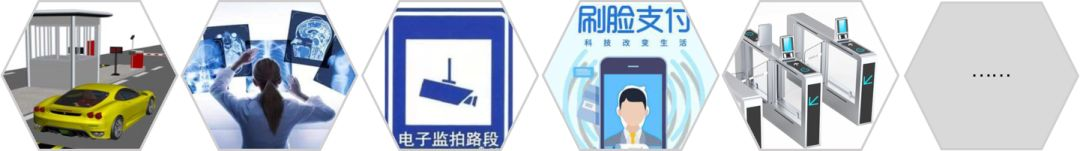• 卷积神经网络：卷积神经网络（Convolutional Neural Networks, CNN）是计算机视觉技术最经典的模型结构。这里主要介绍卷积神经网络的常用模块，包括：卷积、池化等。

• 图像分类：介绍图像分类算法的经典模型结构，并通过眼疾筛查的案例展示算法的应用。

• 目标检测：介绍目标检测YOLO-V3算法，并通过林业病虫害数据集中的虫子检测任务案例展示YOLO-V3算法的应用。

03 计算机视觉发展历程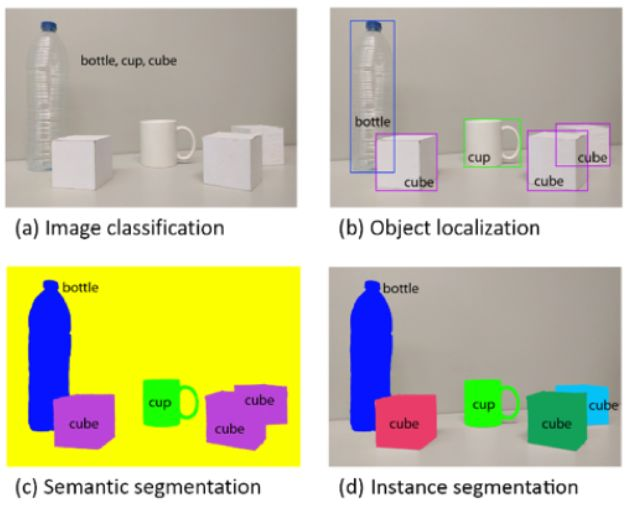• (a) Image Classification： 图像分类，用于识别图像中物体的类别（如：bottle、cup、cube）

• (b) Object Localization： 目标检测，用于检测图像中每个物体的类别，并准确标出它们的位置。

• (c) Semantic Segmentation： 图像语义分割，用于标出图像中每个像素点所属的类别，属于同一类别的像素点用一个颜色标识。

• (d) Instance Segmentation： 实例分割，值得注意的是，（b）中的目标检测任务只需要标注出物体位置，而（d）中的实例分割任务不仅要标注出物体位置，还需要标注出物体的外形轮廓。

04 卷积神经网络

• 卷积（Convolution）

• 池化（Pooling）

• ReLU激活函数

• 批归一化（Batch Normalization）

• 丢弃法（Dropout）

1. 输入数据的空间信息被丢失。 空间上相邻的像素点往往具有相似的RGB值，RGB的各个通道之间的数据通常密切相关，但是转化成1维向量时，这些信息被丢失。同时，图像数据的形状信息中，可能隐藏着某种本质的模式，但是转变成1维向量输入全连接神经网络时，这些模式也会被忽略。

2. 模型参数过多，容易发生过拟合。 在手写数字识别案例中，每个像素点都要跟所有输出的神经元相连接。当图片尺寸变大时，输入神经元的个数会按图片尺寸的平方增大，导致模型参数过多，容易发生过拟合。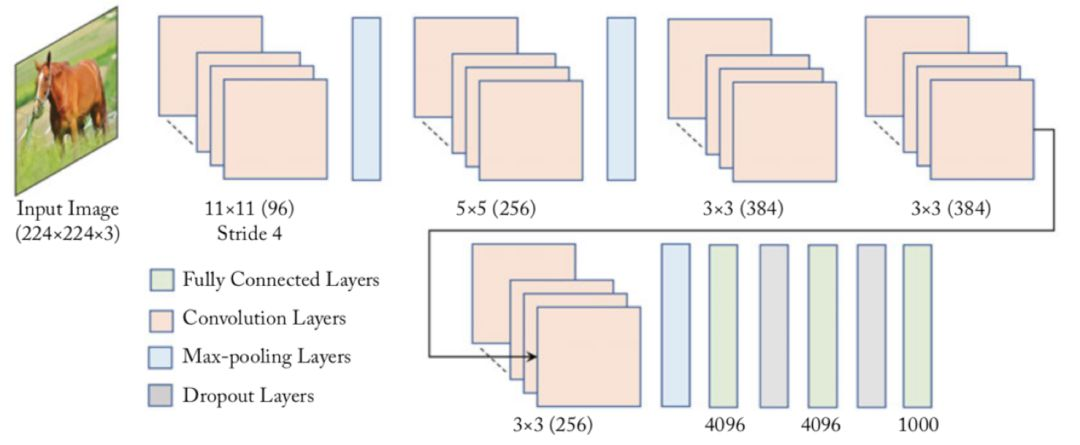05 卷积（Convolution）

• 卷积计算

• 填充（padding）

• 步幅（stride）

• 感受野（Receptive Field）

• 多输入通道、多输出通道和批量操作

• 飞桨卷积API介绍

• 卷积算子应用举例

### 卷积计算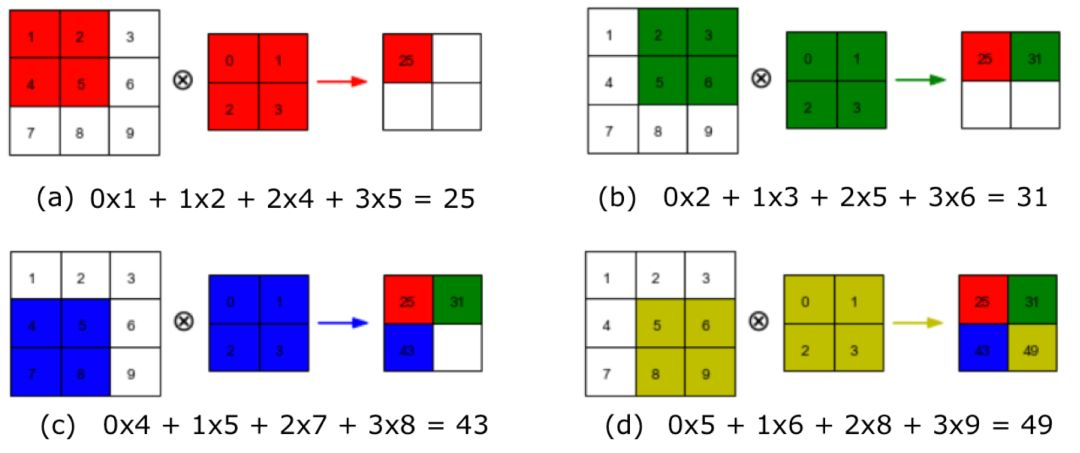• 如图4（a）所示：左边的图大小是，表示输入数据是一个维度为的二维数组；中间的图大小是，表示一个维度为的二维数组，我们将这个二维数组称为卷积核。先将卷积核的左上角与输入数据的左上角（即：输入数据的(0, 0)位置）对齐，把卷积核的每个元素跟其位置对应的输入数据中的元素相乘，再把所有乘积相加，得到卷积输出的第一个结果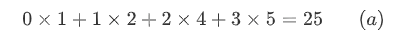• 如图4（b）所示：将卷积核向右滑动，让卷积核左上角与输入数据中的(0,1)位置对齐，同样将卷积核的每个元素跟其位置对应的输入数据中的元素相乘，再把这4个乘积相加，得到卷积输出的第二个结果，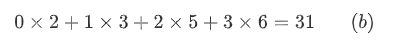• 如图4（c）所示：将卷积核向下滑动，让卷积核左上角与输入数据中的(1, 0)位置对齐，可以计算得到卷积输出的第三个结果，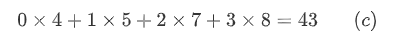• 如图4（d）所示：将卷积核向右滑动，让卷积核左上角与输入数据中的(1, 1)位置对齐，可以计算得到卷积输出的第四个结果，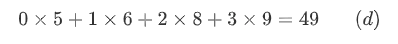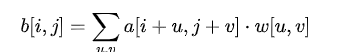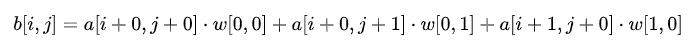• 【思考】 当卷积核大小为时，b和a之间的对应关系应该是怎样的？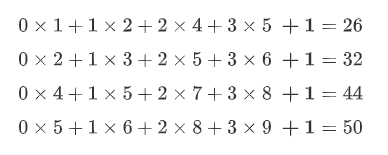### 填充（padding）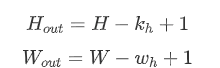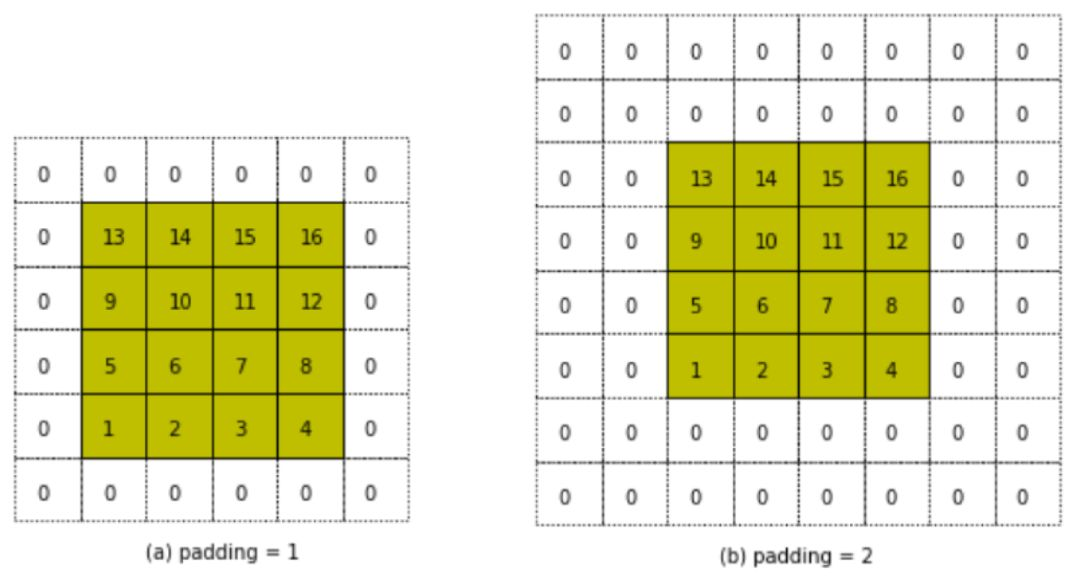• 如图5（a）所示：填充的大小为1，填充值为0。填充之后，输入图片尺寸从变成了，使用3x3的卷积核，输出图片尺寸为。

• 如图5（b）所示：填充的大小为2，填充值为0。填充之后，输入图片尺寸从变成了，使用3x3的卷积核，输出图片尺寸为。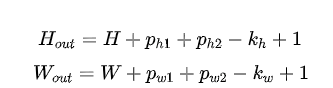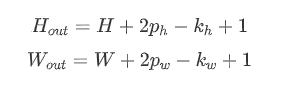### 步幅（stride）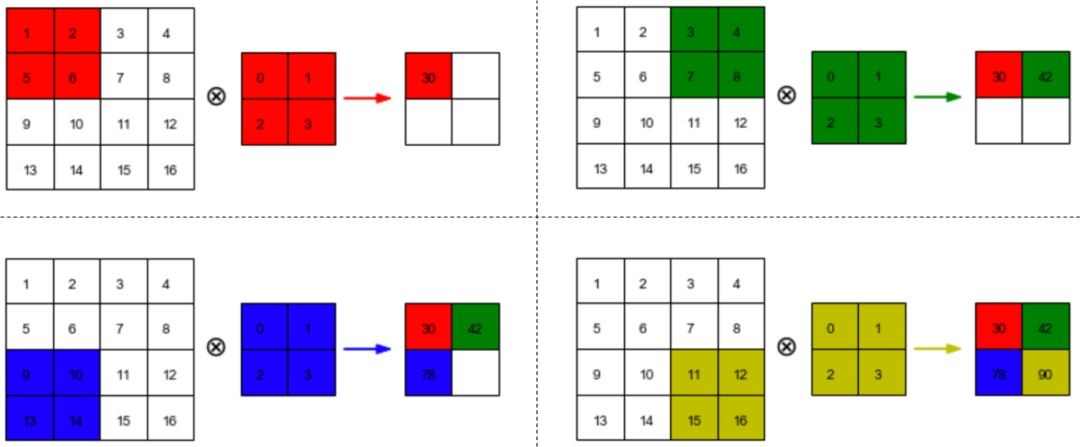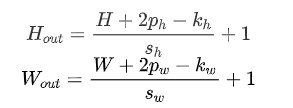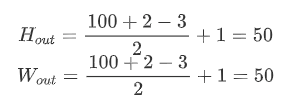### 多输入通道、多输出通道和批量操作

• 多输入通道场景

1. 对每个通道分别设计一个2维数组作为卷积核，卷积核数组的形状是。

2. 对任一通道，分别用大小为的卷积核在大小为的二维数组上做卷积。

3. 将这个通道的计算结果相加，得到的是一个形状为的二维数组。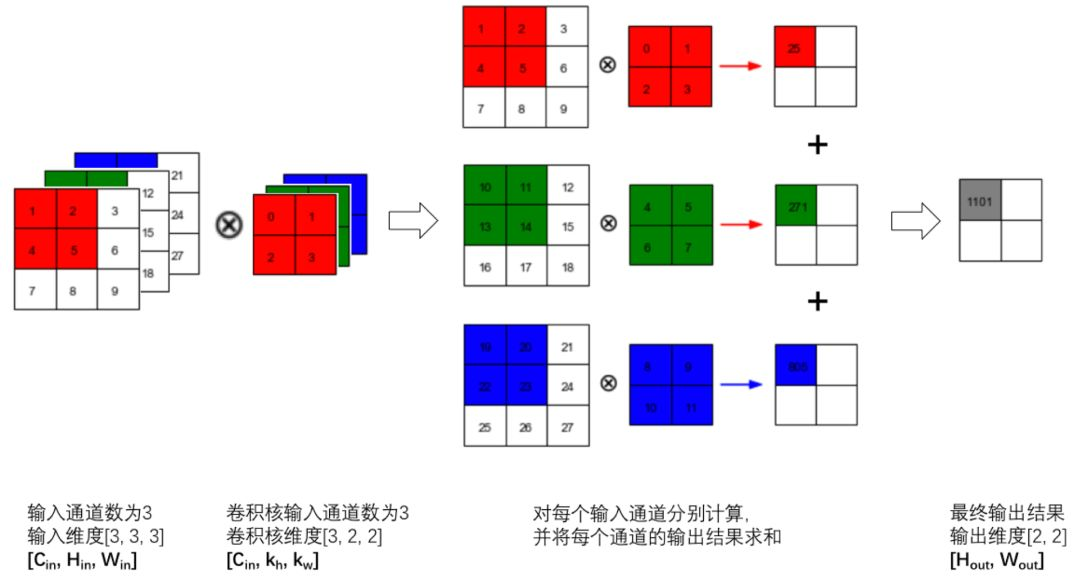p='gray')     f =

import matplotlib.pyplot as plt
import numpy as npimport paddleimport paddle.fluid as fluidfrom paddle.fluid.dygraph.nn import Conv2Dfrom paddle.fluid.initializer import NumpyArrayInitializer%matplotlib inline
with fluid.dygraph.guard(): # 创建初始化权重参数w w = np.array([1, 0, -1], dtype='float32') # 将权重参数调整成维度为[cout, cin, kh, kw]的四维张量 w = w.reshape([1, 1, 1, 3]) # 创建卷积算子，设置输出通道数，卷积核大小，和初始化权重参数 # filter_size = [1, 3]表示kh = 1, kw=3 # 创建卷积算子的时候，通过参数属性param_attr，指定参数初始化方式 # 这里的初始化方式时，从numpy.ndarray初始化卷积参数 conv = Conv2D('conv', num_filters=1, filter_size=[1, 3], param_attr=fluid.ParamAttr( initializer=NumpyArrayInitializer(value=w)))
# 创建输入图片，图片左边的像素点取值为1，右边的像素点取值为0 img = np.ones([50,50], dtype='float32') img[:, 30:] = 0. # 将图片形状调整为[N, C, H, W]的形式 x = img.reshape([1,1,50,50]) # 将numpy.ndarray转化成paddle中的tensor x = fluid.dygraph.to_variable(x) # 使用卷积算子作用在输入图片上 y = conv(x) # 将输出tensor转化为numpy.ndarray out = y.numpy()
f = plt.subplot(121)f.set_title('input image', fontsize=15)plt.imshow(img, cmap='gray')
f = plt.subplot(122)f.set_title('output featuremap', fontsize=15)# 卷积算子Conv2D输出数据形状为[N, C, H, W]形式# 此处N, C=1，输出数据形状为[1, 1, H, W]，是4维数组# 但是画图函数plt.imshow画灰度图时，只接受2维数组# 通过numpy.squeeze函数将大小为1的维度消除plt.imshow(out.squeeze(), cmap='gray')plt.show()

import matplotlib.pyplot as pltfrom PIL
import Imageimport numpy as npimport paddleimport paddle.fluid as fluidfrom paddle.fluid.dygraph.nn
import Conv2Dfrom paddle.fluid.initializer
import NumpyArrayInitializerimg = Image.open('./work/images/p1/000000098520.jpg') with fluid.dygraph.guard(): #设置卷积核参数 w = np.array([
[-1, -1, -1],
[-1, 8, -1],
[-1, -1, -1]
], dtype = 'float32') / 8 w = w.reshape([1, 1, 3, 3])# 由于输入通道数是3， 将卷积核的形状从[1, 1, 3, 3] 调整为[1, 3, 3, 3] w = np.repeat(w, 3, axis = 1)# 创建卷积算子， 输出通道数为1， 卷积核大小为3x3，# 并使用上面的设置好的数值作为卷积核权重的初始化参数 conv = Conv2D('conv', num_filters = 1, filter_size = [3, 3], param_attr = fluid.ParamAttr(initializer = NumpyArrayInitializer(value = w)))# 将读入的图片转化为float32类型的numpy.ndarray x = np.array(img).astype('float32')# 图片读入成ndarry时， 形状是[H, W, 3]，# 将通道这一维度调整到最前面 x = np.transpose(x, (2, 0, 1))# 将数据形状调整为[N, C, H, W] 格式 x = x.reshape(1, 3, img.height, img.width) x = fluid.dygraph.to_variable(x) y = conv(x) out = y.numpy() plt.figure(figsize = (20, 10)) f = plt.subplot(121) f.set_title('input image', fontsize = 15) plt.imshow(img) f = plt.subplot(122) f.set_title('output feature map', fontsize = 15) plt.imshow(out.squeeze(), cmap = 'gray') plt.show()

import matplotlib.pyplot as plt
from PIL import Image
import numpy as npimport paddleimport paddle.fluid as fluidfrom paddle.fluid.dygraph.nn import Conv2Dfrom paddle.fluid.initializer import NumpyArrayInitializer
# 读入图片并转成numpy.ndarray#img = Image.open('./images/section1/000000001584.jpg')img = Image.open('./work/images/section1/000000355610.jpg').convert('L')img = np.array(img)
# 换成灰度图
with fluid.dygraph.guard(): # 创建初始化参数 w = np.ones([1, 1, 5, 5], dtype = 'float32')/25 conv = Conv2D('conv', num_filters=1, filter_size=[5, 5],  param_attr=fluid.ParamAttr( initializer=NumpyArrayInitializer(value=w)))
x = img.astype('float32') x = x.reshape(1,1,img.shape, img.shape) x = fluid.dygraph.to_variable(x) y = conv(x) out = y.numpy()
plt.figure(figsize=(20, 12))f = plt.subplot(121)f.set_title('input image')plt.imshow(img, cmap='gray')
f = plt.subplot(122)f.set_title('output feature map')out = out.squeeze()plt.imshow(out, cmap='gray')
plt.show()


07 总结

【如何学习】

1. 如何观看配套视频？如何代码实践？

https://aistudio.baidu.com/aistudio/course/introduce/888

1. 学习过程中，有疑问怎么办？

1. 如何学习更多内容？

下载安装命令

## CPU版本安装命令
pip install -f https://paddlepaddle.org.cn/pip/oschina/cpu paddlepaddle

## GPU版本安装命令
pip install -f https://paddlepaddle.org.cn/pip/oschina/gpu paddlepaddle-gpu>> 访问 PaddlePaddle 官网，了解更多相关内容

0
0 收藏

### 作者的其它热门文章0 评论
0 收藏
0